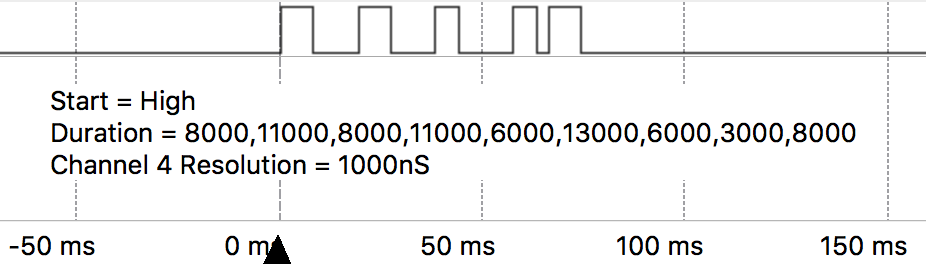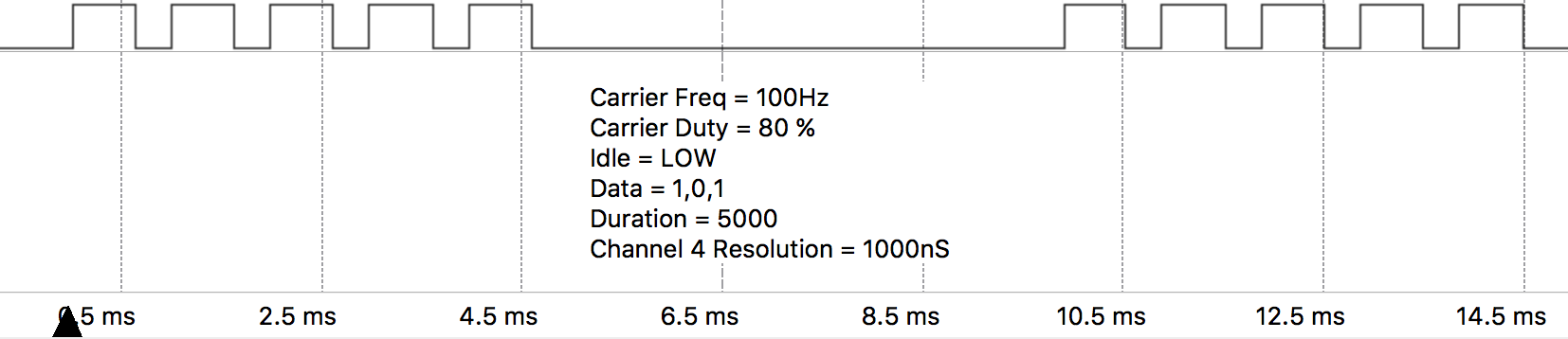toc RMT more_vert What's in this document more_vert Tutorials & Examples > All Pycom Device Examples > RMT

# RMT

Detailed information about this class can be found in `RMT`.

The RMT (Remote Control) peripheral of the ESP32 is primarily designed to send and receive infrared remote control signals that use on-off-keying of a carrier frequency, but due to its design it can be used to generate various types of signals, this class will allow you to do this.

The RMT has 7 channels, of which 5 are available and can be mapped to any GPIO pin (Note: Pins `P13` -`P18` can only be used as inputs).

Channel Resolution Maximum Pulse Width
0 Used by on-board LED
1 Used by `pycom.pulses_get()`
2 100nS 3.2768 ms
3 100nS 3.2768 ms
4 1000nS 32.768 ms
5 1000nS 32.768 ms
6 3125nS 102.4 ms
7 3125nS 102.4 ms

## Transmitting

The following examples create an RMT object on channel 4, configure it for transmission and send some data in various forms. The resolution of channel 4 is 1000 nano seconds, the given values are interpreted accordingly.

In this first example, we define the signal as a tuple of binary values that define the shape of the desired signal along with the duration of a bit.

``````from machine import RMT
# Map RMT channel 4 to P21, when the RMT is idle, it will output LOW
rmt = RMT(channel=4, gpio="P21", tx_idle_level=RMT.LOW)

# Produces the pattern shown in data, where each bit lasts
# duration * channel resolution = 10000 * 1000ns = 10ms
data = (1,0,1,1,1,0,1,0,1)
duration = 10000
rmt.pulses_send(duration, data)``````In this example we define the signal by a tuple of durations and what state the signal starts in.

``````from machine import RMT
# Map RMT channel 4 to P21, when the RMT is idle, it will output LOW
rmt = RMT(channel=4, gpio="P21", tx_idle_level=RMT.LOW)

# The list of durations for each pulse to be, these are in units of the channels
# resolution:
#    duration = Desired pulse length / Channel Resolution
duration = (8000,11000,8000,11000,6000,13000,6000,3000,8000)

# `start_level` defines if the signal starts off as LOW or HIGH, it will then
# toggle state between each duration
rmt.pulses_send(duration, start_level=RMT.HIGH)``````This third example, is a combination of the above two styles of defining a signal. Each pulse has a defined duration as well as a state. This is useful if you don’t always want the signal to toggle state.

``````from machine import RMT
# Map RMT channel 4 to P21, when the RMT is idle, it will output LOW
rmt = RMT(channel=4, gpio="P21", tx_idle_level=RMT.LOW)

# Produces the pattern shown in data, where each bit lasts
# duration[i] * channel resolution = duration[i] * 1000ns
data = (1,0,1,1,0,1)
duration = (400,200,100,300,200,400)
rmt.pulses_send(duration, data)``````The following example creates an RMT object on channel 4 and configures it for transmission with carrier modulation.

``````from machine import RMT
rmt = RMT(channel=4,
gpio="P21",
tx_idle_level=RMT.LOW,
# Carrier = 100Hz, 80% duty, modules HIGH signals
tx_carrier = (100, 70, RMT.HIGH))
data = (1,0,1)
duration = 10000
rmt.pulses_send(duration, data)``````The following example creates an RMT object on channel 2, configures it for receiving, then waits for the first, undefined number of pulses without timeout

``````from machine import RMT
rmt = machine.RMT(channel=2)
rmt.init(gpio="P21", rx_idle_threshold=1000)

data = rmt.pulses_get()``````
``````If tx_idle_level is not set to the opposite of the third value in the tx_carrier tuple, the carrier wave will continue to be generated when the RMT channel is idle.
``````

## Receiving

The following example creates an RMT object on channel 2, configures it for receiving a undefined number of pulses, then waits maximum of 1000us for the first pulse.

``````from machine import RMT
# Sets RMT channel 2 to P21 and sets the maximum length of a valid pulse to
# 1000*channel resolution = 1000 * 100ns = 100us
rmt = machine.RMT(channel=2, gpio="P21", rx_idle_threshold=1000)
rmt.init()

# Get a undefined number of pulses, waiting a maximum of 500us for the first
# pulse (unlike other places where the absolute duration was based on the RMT
# channels resolution, this value is in us) until a pulse longer than
# rx_idle_threshold occurs.
data = rmt.pulses_get(timeout=500)``````

The following example creates an RMT object on channel 2, configures it for receiving, filters out pulses with width < 20*100 nano seconds, then waits for 100 pulses

``````from machine import RMT

rmt = machine.RMT(channel=2,  # Resolution = 100ns
gpio="P21",
# Longest valid pulse = 1000*100ns = 100us
rx_idle_threshold=1000,
# Filter out pulses shorter than 20*100ns = 2us
rx_filter_threshold=20)

# Receive 100 pulses, pulses shorter than 2us or longer than 100us will be
# ignored. That means if it receives 80 valid pulses but then the signal
# doesn't change for 10 hours and then 20 more pulses occur, this function
# will wait for 10h
data = rmt.pulses_get(pulses=100)``````

Previous Next